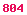# Markov Functional Equity Model

Fries, Christian P.: Markov Functional Modeling of Equity, Commodity and other Assets. Version 0.8.1. March 31, 2006. [276 KB].

### Contents

 1 Introduction 3 2 Markov Functional Model with Asset Numéraire 3 2.1 Markov Functional Assumption 3 3 Example: The Black-Scholes Model 4 4 Numerical Calibration to a Full Two Dimensional European Option Smile Surface 5 4.1 Market Price 5 4.2 Model Price 5 4.3 Solving for the Functional 6 5 Interest Rates 6 5.1 A Note on Interest Rates and the No-Arbitrage Requirement 6 5.2 Where are the Interest Rates? 6 6 Model Dynamics 7 6.1 Introduction 7 6.1.1 Time Copula 8 6.1.2 Time-Discrete Markovian Driver 8 6.2 Interest Rate Dynamics 8 6.2.1 Example: Black-Scholes Model with a Term Structure of Volatility 8 6.2.2 Calibration to arbitrary Interest Rate Dynamics 10 6.3 Forward Volatility 10 6.3.1 Example: Black-Scholes Model with a Term Structure of Volatility 10 6.3.2 Example: Exponential Decaying Instantaneous Volatility 11 7 Implementation 11 7.1 Calibration of the Functional Forms 11 8 Conclusion 13 List of Symbols 14 References 15 Notes 15

### Abstract

In this short note we show how to setup a one dimensional single asset model, e.g. equity model, which calibrates to a full (two dimensional) implied volatility surface. We show that the efficient calibration procedure used in LIBOR Markov functional models may be applied here too. In a addition to the calibration to a full volatility surface the model allows the calibration of the joint asset-interest rate movement (i.e. local interest rates) and forward volatility. The latter allows the calibration of compound or Bermudan options.

The Markov functional modeling approach consists of a Markovian driver process x and a mapping functional representing the asset states S(t) as a function of x(t). It was originally developed in the context of interest rate models, see [Hunt Kennedy Pelsser 2000]. Our approach however is similar to the setup of the hybrid Markov functional model in spot measure, as considered in [Fries Rott 2004].

For equity models it is common to use a deterministic Numéraire, e.g. the bank account with deterministic interest rates. In our approach we will choose the asset itself as Numéraire. This is a subtle, but crucial difference to other approaches considering Markov functional modeling. Choosing the asset itself as Numéraire will allow for a very efficient numerically calibration procedure. As a consequence interest rates have to be allowed to be stochastic, namely as a functional of x too. The Black-Scholes model with deterministic interest rates is a special case of such a Markov functional model.

The most general form of this modeling approach will allow for a simultaneous calibration to a full two dimensional volatility smile, a prescribed joint movement of interest rates and a given forward volatility structure.

### Classification

• MSC-class: 65C05 (Primary), 68U20, 60H35 (Secondary).
• ACM-class: G.3; I.6.8.
• JEL-class: C15, G13.
• Keywords: Markov functional model, implied modeling, equity, commodity, lattice model.

Comments and suggestions welcomed at email@christian-fries.de.